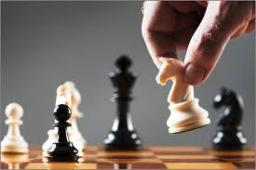# Representing 5562

The chess group attends eight boys, representing 40% of all group members. How many girls go to the chess ring?

d =  12

### Step-by-step explanation:

8 = 40/100 • (8+d)

40d = 480

d = 12

Our simple equation calculator calculates it.Did you find an error or inaccuracy? Feel free to write us. Thank you!

Tips for related online calculators
Need help calculating sum, simplifying, or multiplying fractions? Try our fraction calculator.